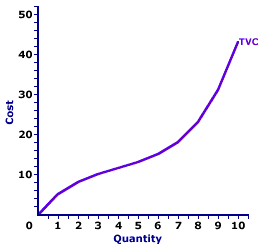Saturday  November 28, 2020
 AmosWEB means Economics with a Touch of Whimsy!DIVIDEND: The portion of a corporation's after-tax accounting profit that's paid to shareholders or owners. Corporate managers usually try to pay the shareholders some minimum dividend that's comparable to returns from other financial markets--such as the interest on government securities or corporate bonds--to keep the owners from selling off the company's stock. That portion of after-tax accounting profit that's not paid out as dividends is typically invested in capital.TOTAL VARIABLE COST AND MARGINAL COST:

A mathematical connection between marginal cost and total variable cost stating that marginal cost IS the slope of the total variable cost curve. This relation between total variable cost and marginal cost is also seen with total cost. The slope of the total cost curve is marginal cost, as well. The relation between total variable cost and marginal cost is but another in the long line of applications of the total-marginal relation.
Total Variable Cost and Marginal CostThe slope of the total variable cost curve (and total cost curve) is marginal cost. As such, if the total variable cost curve has a positive slope (that is, is upward sloping), then marginal cost is positive. Moreover, if the total variable cost curve has a positive and increasingly steeper slope, then the marginal cost is positive and rising. If the total variable cost curve has a positive and increasingly flatter slope, then the marginal cost is positive but falling.

This particular total-marginal relation applies to both total variable cost and total cost. Because not only is marginal cost the slope of the total variable cost curve, it is also the slope of the total cost curve. The reason is that any changes in total cost resulting from changing output is matched by changes in total variable cost. This occurs because total fixed cost is FIXED.

This two-paneled graph for the production of Wacky Willy Stuffed Amigos (those cute and cuddly armadillos, turtles, and lizards) visually illustrates the connection between total variable cost and marginal cost. For the first few quantities of output (Stuffed Amigos), total variable cost in the top panel is positive AND the slope of the total variable cost curve decreases--it becomes flatter. This corresponds with a positive and decreasing marginal cost in the bottom panel up to 4 Stuffed Amigos. For the next several quantities of Stuffed Amigos output, the slope of the total variable cost curve becomes increasingly steeper. This corresponds to an increasing marginal cost in the bottom panel.

The prime conclusion is the key role played by the law of diminishing marginal returns in the slope of both the marginal cost curve and the total variable cost curve.

• The U-shape of the marginal cost curve is a direct reflection of first increasing marginal returns, as marginal cost falls to a minimum, then decreasing marginal returns and the onset of the law of diminishing marginal returns as marginal cost rises.

• However, because the marginal cost curve is a plot of the slope of the total variable cost curve, then the shape of the total variable cost curve also reflects the law of diminishing marginal returns. The flattening slope of the total variable cost curve for small quantities of output is due to increasing marginal returns. Then the onset of the law of diminishing marginal returns causes the total variable cost curve to become increasingly steeper.
While this diagram relates marginal cost and total variable cost, the same story applies to the relation between marginal cost and total cost. Marginal cost is also the slope of the total cost curve. As such, the shape of the total cost curve is also a reflection of increasing, then decreasing marginal returns.

 <= TOTAL VARIABLE COST TOTAL VARIABLE COST AND TOTAL PRODUCT =>Recommended Citation:

TOTAL VARIABLE COST AND MARGINAL COST, AmosWEB Encyclonomic WEB*pedia, http://www.AmosWEB.com, AmosWEB LLC, 2000-2020. [Accessed: November 28, 2020].

Check Out These Related Terms...

Or For A Little Background...

And For Further Study...
Search Again?BLUE PLACIDOLA[What's This?] Today, you are likely to spend a great deal of time surfing the Internet looking to buy either clothing for your pet dog or an ink cartridge for your printer. Be on the lookout for poorly written technical manuals.Your Complete ScopeThe average bank teller loses about \$250 every year."The world is not dangerous because of those who do harm but because of those who look at it without doing anything. "-- Albert Einstein, physicistMBAMaster of Business AdministrationA PEDestrian's Guide Xtra CreditTell us what you think about AmosWEB. Like what you see? Have suggestions for improvements? Let us know. Click the User Feedback link.| | | | | | | | | | |
| | | |

Thanks for visiting AmosWEB